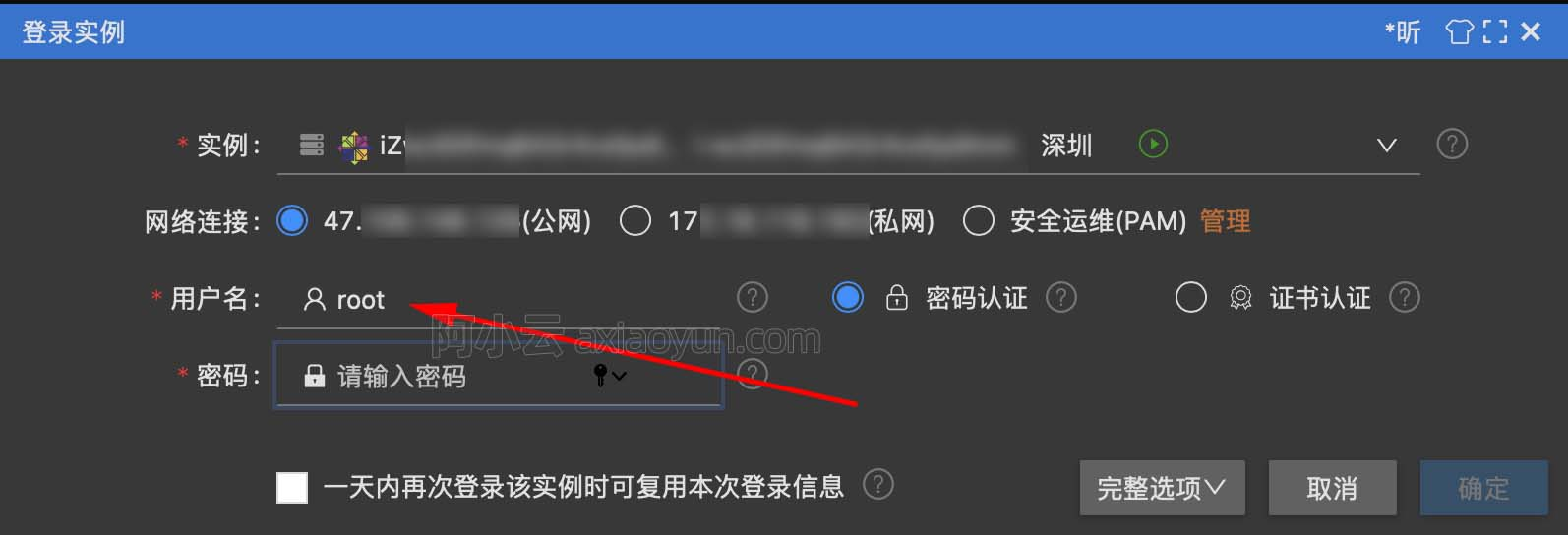PyODPS开发中的最佳实践

+关注继续查看

PyODPS 支持用 Python 来对 MaxCompute 对象进行操作，它提供了 DataFrame API 来用类似 pandas 的接口进行大规模数据分析以及预处理，并且可以用 ml 模块来执行机器学习算法。

除非数据量很小，否则不要试图进行本地数据处理

In : df
json
0  {"a": 1, "b": 2}
1  {"c": 4, "b": 3}

In : from odps.df import output

In : @output(['k', 'v'], ['string', 'int'])
...: def h(row):
...:     import json
...:     for k, v in json.loads(row.json).items():
...:         yield k, v
...:

In : df.apply(h, axis=1)
k  v
0  a  1
1  b  2
2  c  4
3  b  3

使用 pandas 计算后端进行高效本地 debug

PyODPS DataFrame 能够根据数据来源来决定如何执行，比如，通过 pandas DataFrame 创建的 PyODPS DataFrame 则可以使用 pandas 执行本地计算；而使用 MaxCompute 表创建的 DataFrame 则可以在 MaxCompute 上执行。 而这两种方式，除了初始化不同，后续代码完全一致，因此，我们可以利用这点来进行本地 debug。

df = o.get_table('movielens_ratings').to_df()
DEBUG = True
if DEBUG:
df = df[:100].to_pandas(wrap=True)

to_pandas 是将数据下载，根据 wrap 参数来决定是否返回 PyODPS DataFrame，如果是 True，则返回 PyODPS DataFrame；否则，返回 pandas DataFrame。

利用 Python 语言特性来实现丰富的功能

编写 Python 函数

def euclidean_distance(from_x, from_y, to_x, to_y):
return ((from_x - to_x) ** 2 + (from_y - to_y) ** 2).sqrt()

def manhattan_distance(center_x, center_y, x, y):
return (from_x - to_x).abs() + (from_y - to_y).abs()

In : df
from_x    from_y      to_x      to_y
0  0.393094  0.427736  0.463035  0.105007
1  0.629571  0.364047  0.972390  0.081533
2  0.460626  0.530383  0.443177  0.706774
3  0.647776  0.192169  0.244621  0.447979
4  0.846044  0.153819  0.873813  0.257627
5  0.702269  0.363977  0.440960  0.639756
6  0.596976  0.978124  0.669283  0.936233
7  0.376831  0.461660  0.707208  0.216863
8  0.632239  0.519418  0.881574  0.972641
9  0.071466  0.294414  0.012949  0.368514

In : euclidean_distance(df.from_x, df.from_y, df.to_x, df.to_y).rename('distance')
distance
0  0.330221
1  0.444229
2  0.177253
3  0.477465
4  0.107458
5  0.379916
6  0.083565
7  0.411187
8  0.517280
9  0.094420

In : manhattan_distance(df.from_x, df.from_y, df.to_x, df.to_y).rename('distance')
distance
0  0.392670
1  0.625334
2  0.193841
3  0.658966
4  0.131577
5  0.537088
6  0.114198
7  0.575175
8  0.702558
9  0.132617

利用 Python 语言的条件和循环语句

table_names = ['table1', ..., 'tableN']
dfs = [o.get_table(tn).to_df() for tn in table_names]
reduce(lambda x, y: x.union(y), dfs)

df = dfs
for other_df in dfs[1:]:
df = df.union(other_df)

尽量使用内建算子，而不是自定义函数

In : euclidean_distance(df.from_x, df.from_y, df.to_x, df.to_y).rename('distance').mean()
|==========================================|   1 /  1  (100.00%)         7s
0.5216082314224464

In : @output(['distance'], ['float'])
...: def euclidean_distance2(row):
...:     import math
...:     return math.sqrt((row.from_x - row.to_x) ** 2 + (row.from_y - row.to_y) ** 2)
...:

In : df.apply(euclidean_distance2, axis=1, reduce=True).mean()
|==========================================|   1 /  1  (100.00%)        27s
0.5216082314224464

总结10072 06763 0Xshell使用SSH远程登录阿里云ECS服务器CentOS7
13108 013882 011888 0MaxCompute（原ODPS）开发入门指南——数据开发工具篇

6397 0MaxCompute（原ODPS）开发入门指南——计量计费篇

6041 04502 07363 054761 0+关注

28

6

MaxCompute（原ODPS）是一项面向分析的大数据计算服务，它以Serverless架构提供快速、全托管的在线数据仓库服务，消除传统数据平台在资源扩展性和弹性方面的限制，最小化用户运维投入，使您经济并高效的分析处理海量数据。
+ 订阅

《2021云上架构与运维峰会演讲合集》

《零基础CSS入门教程》

《零基础HTML入门教程》